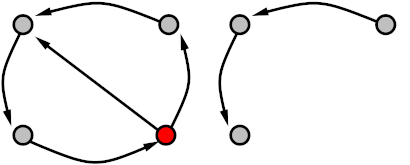# PACE 2022 - Directed Feedback Vertex Set

The Directed Feedback Vertex Set Problem is defined as follows:

Input: A directed graph $G = (V, E)$.
Output: Find a minimum subset $X \subseteq V$ such that, when all vertices of $X$ and their adjacent edges are deleted from $G$, the remainder is acyclic.

Thus a feedback vertex set of a graph is a set of vertices whose deletion leaves a graph acyclic.

### ExampleIn this example, deleting/removing the red vertex and its edges in the left graph results in the graph on the right hand side and leaves the remaining graph without any cycles.

### Background

The Directed Feedback Vertex Set Problem has a wide range of applications including deadlock detection, program verification and VLSI chip design. The problem is NP-complete even if restricted to graphs with maximum in- and out-degree two. The optimization problem can be solved in $O^*(1.9977^n)$ due to an algorithm by Razgon. Chen et al. have shown that the problem is fixed-parameter tractable if parameterized with the solution size $k$, i.e. Chen et al. develop an algorithm with running time $4^kk!n^{O(1)}$. Note that the problem is equivalent to the edge-deletion variant commonly called Feedback Arc Set: there are reductions in both ways that preserve the value of the optimal solution and only blow up the size of the graph (sum of vertices and edges) by a polynomial factor.

## Literature

• Cygan, Marek; Fomin, Fedor V.; Kowalik, Lukasz; Lokshtanov, Daniel; Marx, Daniel; Pilipczuk, Marcin; Pilipczuk, Michal; Saurabh, Saket (2015), “Parameterized Algorithms”, Chapter 8.6, Springer.
• Karp, Richard M. (1972), “Reducibility Among Combinatorial Problems”, Proc. Symposium on Complexity of Computer Computations, IBM Thomas J. Watson Res. Center, Yorktown Heights, N.Y., New York: Plenum, pp. 85–103
• Razgon, I. (2007), “Computing minimum directed feedback vertex set in $O^*(1.9977^n)$”, in Proceedings of the 10th Italian Conference on Theoretical Computer Science, World Scientific, pp. 70–81
• Chen, Jianer; Liu, Yang; Lu, Songjian; O’Sullivan, Barry; Razgon, Igor (2008), “A fixed-parameter algorithm for the directed feedback vertex set problem”, Journal of the ACM, 55 (5), Art. 21
• Fleischer, Rudolf, Xi Wu, and Liwei Yuan. “Experimental study of FPT algorithms for the directed feedback vertex set problem.” European Symposium on Algorithms. Springer, Berlin, Heidelberg, 2009.